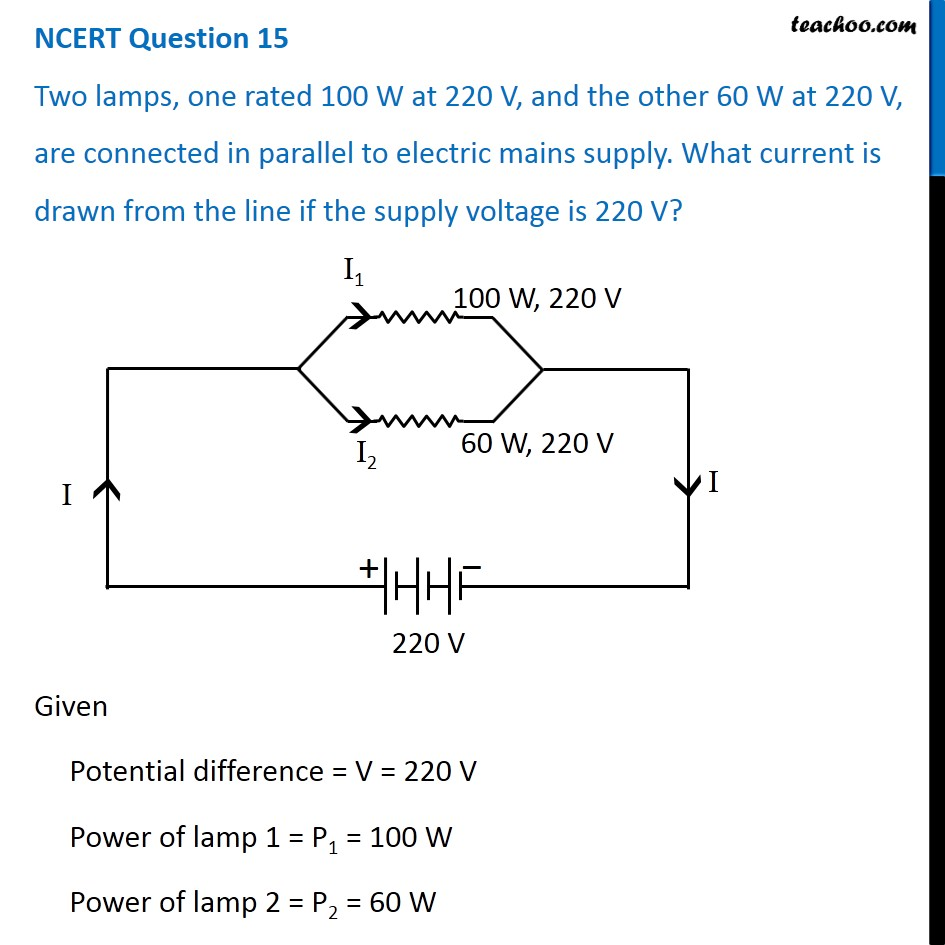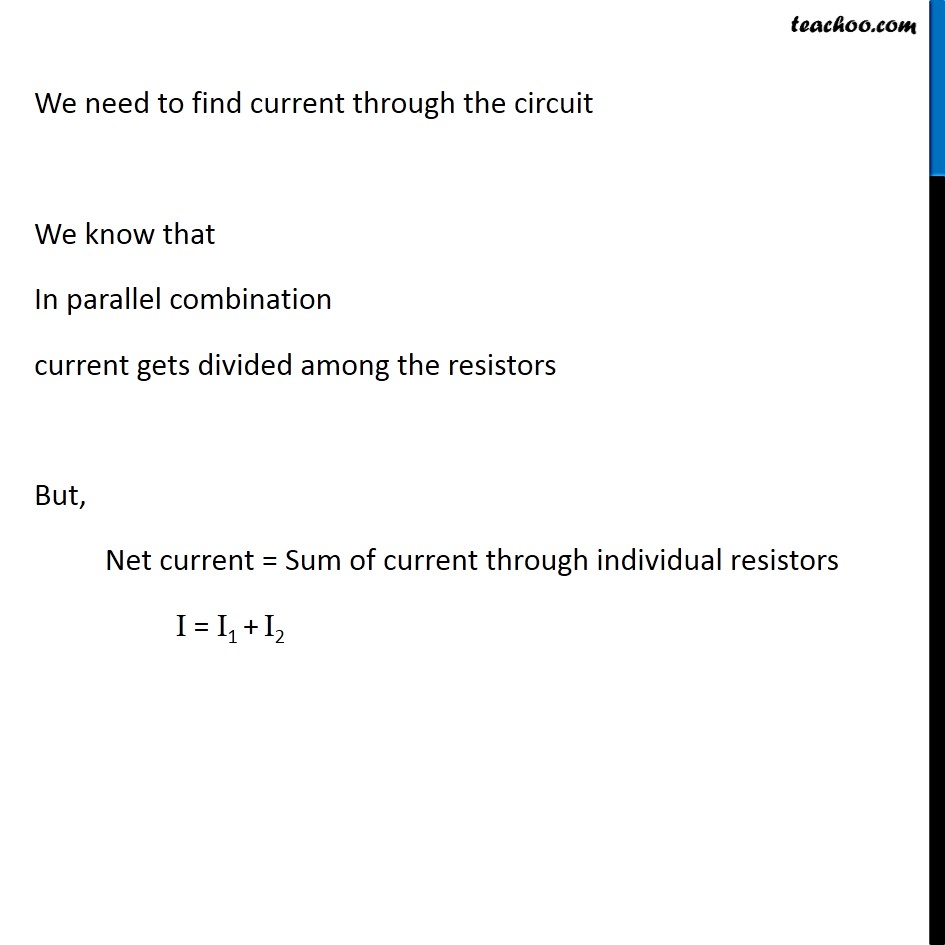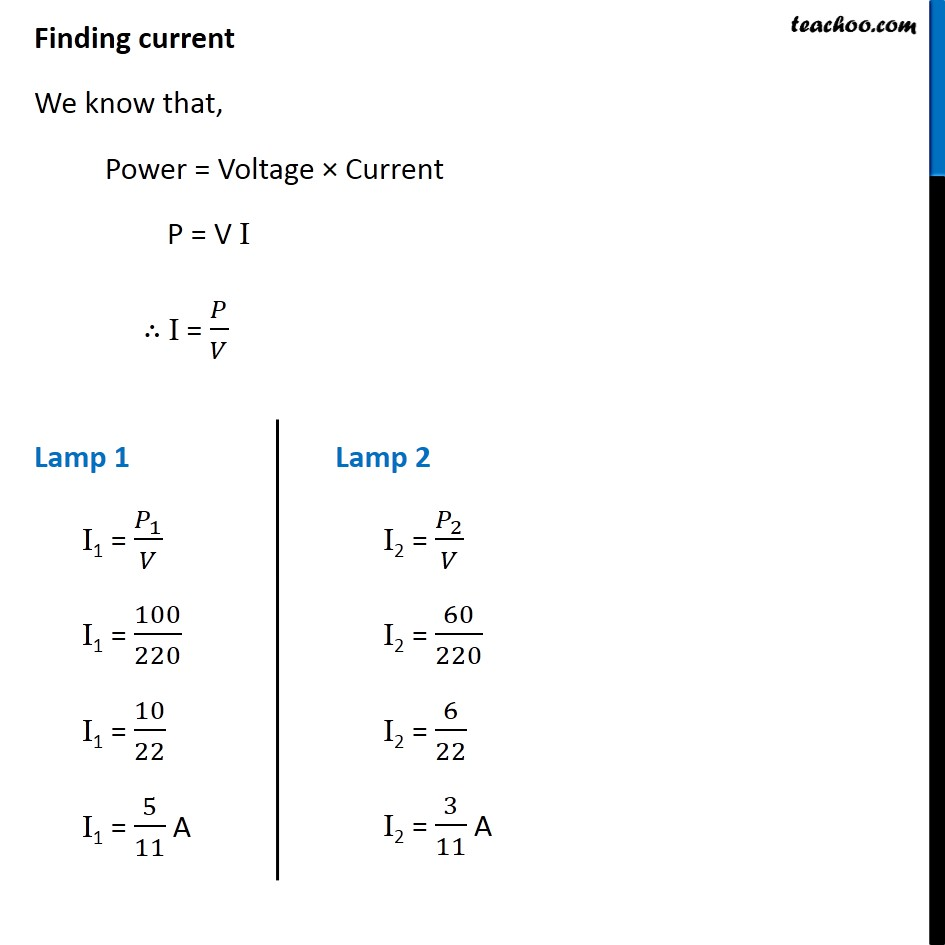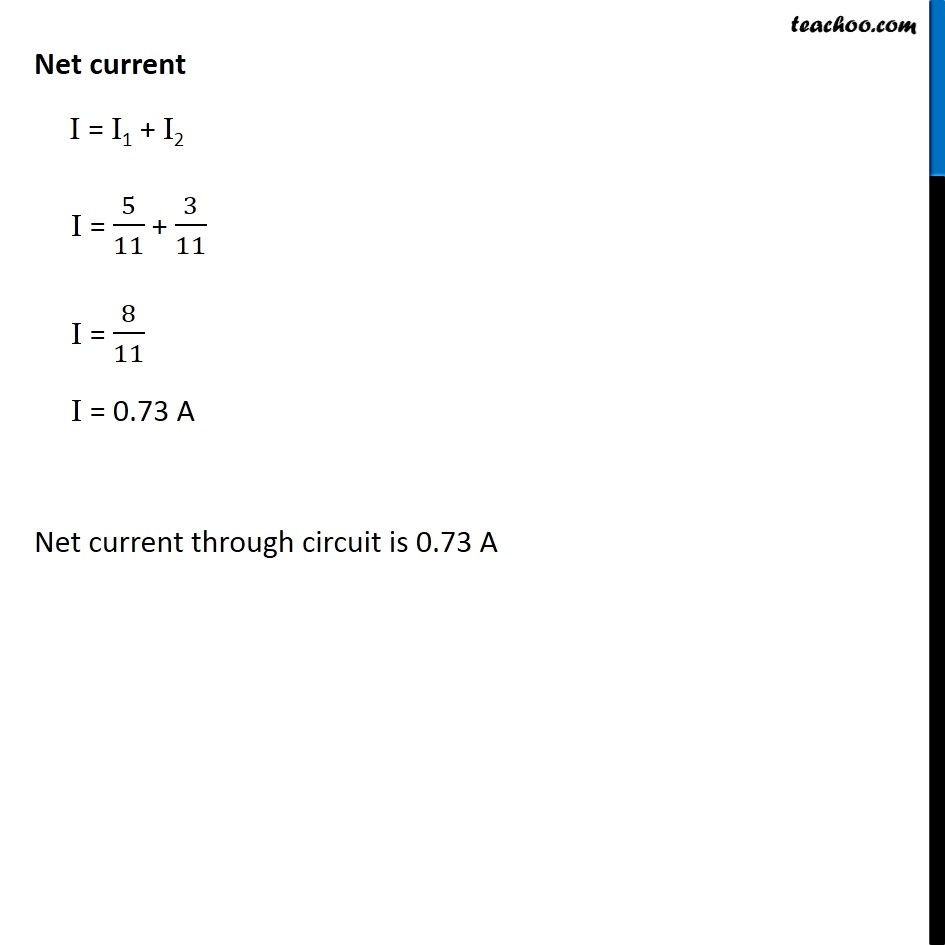NCERT Questions

Class 10
Chapter 12 Class 10 - Electricity

## Two lamps, one rated 100 W at 220 V, and the other 60 W at 220 V, are connected in parallel to electric mains supply. What current is drawn from the line if the supply voltage is 220 V?Learn in your speed, with individual attention - Teachoo Maths 1-on-1 Class

### Transcript

NCERT Question 15 Two lamps, one rated 100 W at 220 V, and the other 60 W at 220 V, are connected in parallel to electric mains supply. What current is drawn from the line if the supply voltage is 220 V? Given Potential difference = V = 220 V Power of lamp 1 = P1 = 100 W Power of lamp 2 = P2 = 60 W We need to find current through the circuit We know that In parallel combination current gets divided among the resistors But, Net current = Sum of current through individual resistors I = I1 + I2 Finding current We know that, Power = Voltage × Current P = V I ∴ I = 𝑃/𝑉 Lamp 1 I1 = 𝑃_1/𝑉 I1 = 100/220 I1 = 10/22 I1 = 5/11 A Lamp 2 I2 = 𝑃_2/𝑉 I2 = 60/220 I2 = 6/22 I2 = 3/11 A Net current I = I1 + I2 I = 5/11 + 3/11 I = 8/11 I = 0.73 A Net current through circuit is 0.73 A